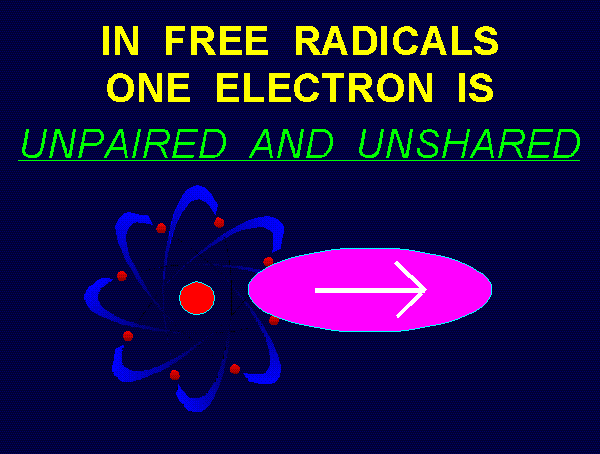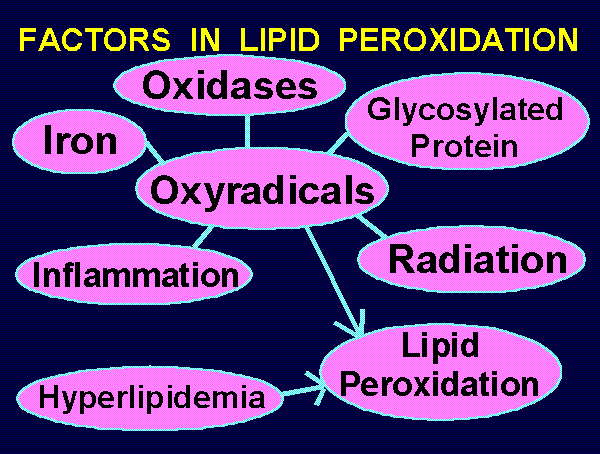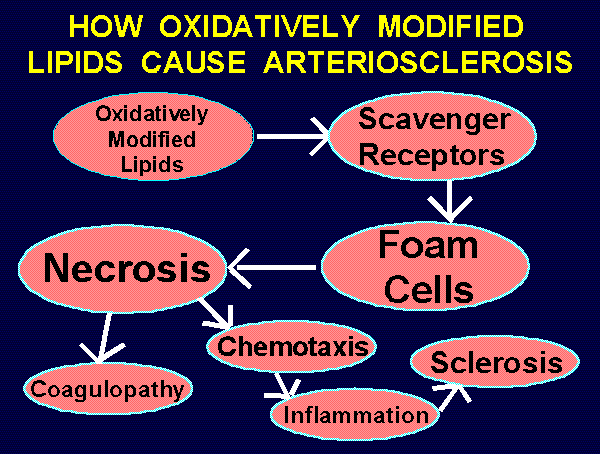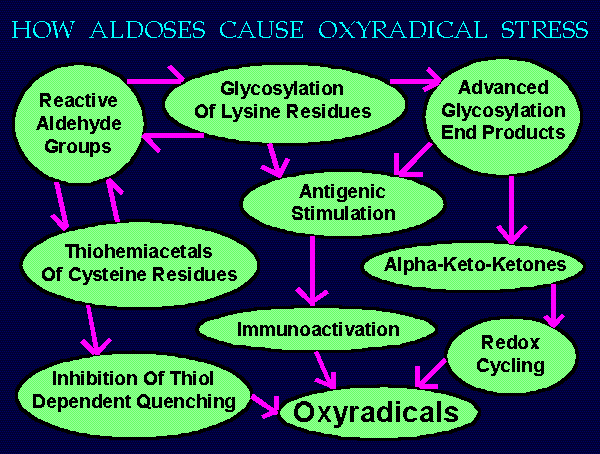HOME~~~PREV~~~NEXT
```		~~~    CHEMICAL  BOND  TYPES    ~~~
Several types of chemical bonds exist which hold
atoms together within and between molecules.
IONIC BONDS
ION DIPOLE ATTRACTIONS
DIPOLE-DIPOLE ATTRACTIONS
LIGAND-CATION COMPLEXES
CHELATE CATION COMPLEXES
HYDROGEN BONDS
METALLIC BONDS
COVALENT BONDS

~~~    COVALENT  BOND  TYPES    ~~~
In covalent bonds two electrons (each of opposite
magnetic polarity or "spin") are paired and shared.BOND TYPES          SYMBOLS:
Sigma               X--X
Sigma & Pi          X==X
Conjugate           X==X--X==XBOUND RADICALS are parts of a molecule which are
covalently bound to the rest of the molecule.
They are often thought of as rays or limbs.
Examples:
methyl groups as in DMSO and dimethyl glycine
O                      CH3     O
||                     |       ||
CH3--S--CH3            CH3--N--CH2--C--OH
hydroxyl  groups  as  in  alcohols  and  phenols
HC==CH
CH3--CH2--OH             /      \
HC        C--OH
\\    //
HC--CH
amino  groups  as  in  urea  and  lysine
O                                HO--C==O
||                                   |
NH2--C--NH2      NH2--CH2--CH2--CH2--CH2--CH--NH2

= "FREE RADICALS" are molecular species possessing
an unpaired electron.
= Neither is this unpaired electron shared
between two atoms as in a covalent bond.
= Free radicals may be thought of as limbs or rays
which were broken away from a covalent molecule.
= The unpaired electron functions like a tiny magnet
seeking to be paired with another electron
of opposite magnetic polarity or "spin".
= Examples of carbon centered free radicals:
H  H                            H  O
"ethyl"    HC--C*           "acetyl"       HC--C*
H  H                            H

HC==CH                          HC==CH
/    \                          /    \
"aryl"   HC      C*        "pyridinyl"   RN     HC*
\\    //                         \    /
HC--CH                          HC==CH~~~     RESONANCE     ~~~
Conjugated pi bonds enable negative charges,
positive charges, and unpaired electrons
to shift positions or resonate.
Resonance delocalizes the effects of the
characteristics being shifted.
Resonance makes conjugated products
more energy favorable.
Resonance allows for reactivity to shift
to each permitted position.
*
X--X==X--X==X--X==X--X==X
*
X==X--X--X==X--X==X--X==X
*
X==X--X==X--X--X==X--X==X
*
X==X--X==X--X==X--X--X==X
*
X==X--X==X--X==X--X==X--X

~~~  REACTIONS OF CARBON CENTERED FREE RADICALS  ~~~
COUPLING:
R*   +   R'*   --->   R--R'
REDUCTION:
R*   +   AOH   --->   RH   +   AO*
OXIDATION:
H  H                    H    H
R--C--C*   +   [O]   --->   C==C  +  H[O]*
H  H                    R    H
R*   +   O2    --->   ROO*
R*   +   X==X  --->   R--X--X*

~~~  OXYGEN  CENTERED  FREE  RADICALS  ~~~
These are generally recognized as dangerous.
hydroxyl = HO*       hydroperoxyl = HOO*
alkoxyl  = RO*       alkylperoxyl = ROO*

~~~ REACTIONS OF OXYGEN CENTERED FREE RADICALS ~~~

HYDROGEN  ABSTRACTION:
RO*   +   AOH   --->   ROH   +   AO*
RO*   +   LH    --->   ROH   +   L*

INITIATION  OF  LIPID  PEROXIDATION:
RO*   +   LH    --->   ROH   +   L*
L*    +   O2    --->   LOO*

RO*   +   X==X  --->   RO--X--X*

INITIATION  OF  POLYMERIZATION:
RO*        +  X==X  --->  RO--X--X*
RO--X--X*  +  Y==Y  --->  RO--X--X--Y--Y*

~~~  LIPID PEROXIDES ARE PRODUCED  ~~~

HCH    HCH    HCH
/ \    / \    / \    +    R*    --->
HC==CH HC==CH

HCH    HC*    HCH
/ \    / \    / \    +    RH
HC==CH HC==CH

1)  INITIATION:     R*   +  LH   -->  RH    +  L*
2)  PROPAGATION:    L*   +  O2   -->  LOO*
LOO* +  LH   -->  LOOH  +  L*
3)  TERMINATION:    LOO* +  L*   -->  LOOL
L*   +  L*   -->  LL
LOO* +  AOH  -->  LOOH  + AO*
4)  ELIMINATION:    LOOH +  2[H] -->  LOH   + H2O

~~~ HOW CONJUGATED DIENES ARE PRODUCED ~~~

1.  An initiator or propagator abstracts hydrogen from an
allylic carbon situated between two double bonds:
H                                 *
--C==C--C--C==C--  +  ROO*  --->  --C==C--C--C==C--  +  ROOH
H  H  H  H  H                     H  H  H  H  H
2.  The adjacent pi bonds permit the unpaired electron to
shift positions and thus be delocalized among 3 sites:
*                           *                           *
--C--C==C--C==C--     --C==C--C--C==C--     --C==C--C==C--C--
H  H  H  H  H         H  H  H  H  H         H  H  H  H  H
(left)                    (middle)                  (right)
3.  Diatomic oxygen can couple with the carbon centered
radical at any one of these three sites:
O*                          O*                          O*
O                           O                           O
--C--C==C--C==C--     --C==C--C--C==C--     --C==C--C==C--C--
H  H  H  H  H         H  H  H  H  H         H  H  H  H  H
4.  Reduction to LOOH and subsequently to LOH yeilds
alpha hydroxy conjugated dienes 2 out of 3 times.
OH                          OH                          OH
O                           O                           O
--C--C==C--C==C--     --C==C--C--C==C--     --C==C--C==C--C--
H  H  H  H  H         H  H  H  H  H         H  H  H  H  H

~~~ ALDEHYDES PRODUCED BY SUBSEQUENT BREAKDOWN OF LOOH ~~~
These products are proinflammatory and cytotoxic.

MALONDIALDEHYDE (MDA):
H  H  H
O==C--C--C==O
H

4-HYDROXY-2,3-TRANS-NONENAL (4HNE):
H H H H H H H   H
HC-C-C-C-C-C-C=C-C=O
H H H H H |   H
OH

THERMAL  CLEAVAGE  OF  PEROXIDES:
HOOH   +   heat   --->   HO*   +   HO*
ROOH   +   heat   --->   RO*   +   HO*
UNIVALENT  REDUCTION  OF  PEROXIDES:
HOOH   +   Fe++   --->   HO*   +   OH-   +   Fe+++
ROOH   +   Fe++   --->   RO*   +   OH-   +   Fe+++
R--CH2*   +   O2  --->   R--CH2--O--O*
UNIVALENT  REDUCTION  OF DIATOMIC  OXYGEN:
Fe++   +   O2   +   H+   --->   Fe+++    +   HOO*
hv   +   X--X   --->   X*    +   e-   +   X+
hv   +   HOH    --->   HO*   +   e-   +   H+
hv   +   X==X   --->   *X--X*
ISCHEMIA - REPERFUSION (let [EC] = electron carriers):
[EC]   +   e-   --->   [EC]e-
[EC]e-  +   O2  --->   [EC]   +   *OO-

~~~ PHYSIOLOGIC  GENERATION  OF  OXYRADICALS ~~~
Macrophages, natural killer cells, and other activated
Some superoxide becomes its more dangerous conjugate acid.
(H+)  +  -OO*  --->  HOO*
Hydroperoxyl radical is further reduced by ascorbate.
2HOO* + HOC=COH ---> 2HOOH + O=C-C=O
Hypochlorite can then be produced by myeloperoxidase.
Cl-  +  HOOH  --->  ClO-  +  HOH
Superoxide or hydrogen peroxide can be oxidized
to become singlet oxygen.
-OO*  +  [O]  --->  O=O  +  [O]-
HOOH  +  HCl0 --->  O=O  +  HOH  +  Cl-  +  H+

~~~ NITRIC OXIDE AS OXIDANT DEFENSE SUBSTANCE ~~~
The enzyme "nitric oxide synthase"  (NOS)  uses
NADPH and arginine to produce nitric oxide  (*NO).
Some of the superoxide (-OO*) produced physiologically
couples with nitric oxide to produce peroxynitric acid,
a powerful cytotoxic agent towards pathogens and tumors.
H+   +   -OO*   +   *NO   --->   HOONO
Peroxynitric acid can readily decompose to release
HOONO   --->   HO*   +   *NO2

~~~ PROOXIDANT ASSAULT WEAPONS OF KILLER CELLS ~~~
Superoxide               -OO*
Singlet Oxygen           O=O
Hydroperoxyl             HOO*
Hydrogen Peroxide        HOOH
Hypochlorous Acid        HClO
Nitric Oxide             *NO
Peroxynitric Acid        HOONO
Hydroxyl                 HO*

~~~ HYDROXYL RADICAL PRODUCTION SEQUENCES ~~~
Hydroxyl radicals can be produced by Fenton's reagent.
HOOH  +  Fe++   --->  OH-  +  HO*  +  Fe+++
Superoxide  can  serve  to  reduce  Fe+++  to  Fe++,
thus  enabling  repetition  of  the  Fenton  reaction.
-OO*  +  Fe+++  <--->  O2  +  Fe++
Numerous other reductants including ascorbic acid and
N-acetyl-L-cysteine can reduce  Fe+++  to  Fe++,
thus  enabling  repetition  of  the  Fenton  reaction.
NALC-SH  +  Fe+++  --->  NALC-S*  +  H+  +  Fe++
Thus freely diffusible cationic iron is a dangerous

~~~ HOW ASCORBIC ACID OR OTHER ENEDIOLS PLUS IRON
PRODUCE BOTH HYDROGEN PEROXIDE AND HYDROXYL RADICALS ~~~
Ascorbic acid readily reduces ferric cations to ferrous.
AAH2  +  Fe+++  --->  *AAH  +  H+  +  Fe++
Ferrous cation reversibly produces superoxide.
O2   +  Fe++   <--->  -OO*  +  Fe+++
Superoxide can be further reduced by ascorbic acid,
or it can dismutate.
-OO* + AAH2 + H+ ---> HOOH  +  *AAH
2 -OO*  +  2 H+  ---> 2 HOOH
Ferrous cation will react with the newly formed H2O2
as in the Fenton reaction.
HOOH  +  Fe++   --->  OH-  +  HO*  +  Fe+++
Deferoxamine is superior to EDTA as an inhibitor
of this process.

Because the following can occur:
*  peroxidize  and  modify  lipids
*  deactivate  enzymes
*  consume  nutrients
*  mutate  genes
*  initiate  carcinogenesis
*  destroy  organelles
*  destroy  cells
*  induce inflammation
*  cause  sclerosis

~~~ DISEASES ASSOCIATED WITH FREE RADICAL STRESS ~~~
*  Iron Toxicity
*  Heavy Metal Toxicity
*  Arthritis
*  Diabetes Mellitus
*  Carcinogenesis
*  Chemical Intolerance
*  Macular Degeneration
*  Arteriosclerosis
*  Neurodegeneration

~~~  TESTS  FOR  OXYRADICAL  STRESS  ~~~
*  total lipid peroxides
*  aldehydes (especially malondialdehyde)
*  thiobarbituric acid reactive substances (TBARS)
*  conjugated dienes
*  breath alkanes
*  hydroxylated salicylate
*  oxidatively modified cholesterols
*  oxidatively modified nucleotides (esp. guanine)
*  serum ascorbate/dehydroascorbate ratio
*  RBC intracellular GSH/GSSG ratio
*  RBC fragility
*  platelet aggregation
*  glycosylated proteins and AGES
*  methionine sulfoxide

~~~  FACTORS  IN  LIPID  PEROXIDATION  ~~~
Iron Excess   --->
Oxidases      --->     Lipid      <--- Hyperlipidemia
Glycosylation --->  Peroxidation
Inflammation  --->~~~   ATHEROGENS   ~~~
Induced Modifications Of Lipids:
*  lipid peroxides
*  aldehydes
*  oxycholesterols
*  lysolecithins

~~~  OXYCHOLESTEROLS  ~~~
These are especially irritating and atherogenic
forms of oxidatively modified cholesterol.
They are produced by oxyradicals acting
within LDL particles and within atheromas.
*  7-beta-hydroperoxycholesterol
*  7-beta-hydroxycholesterol
*  7-ketocholesterol
*  5-alpha-epoxycholesterol
*  6-alpha-epoxycholesterol

~~~ HOW OXIDATIVELY MODIFIED LIPIDS CAUSE ARTERIOSCLEROSIS ~~~
Oxidatively modified lipids --->
Uptake by scavenger receptors --->
Conversion of macrophages to "foam cells" --->
Necrosis of foam cells --->
Coagulopathy & Chemotaxis & Chronic inflammation --->
Sclerosis~~~ HOW ALDOSES CAUSE OXYRADICAL STRESS ~~~
Sugars exist as both opened and closed chain isomers.
When open, an aldehyde group is available for reactions.
During episodes of hyperglycemia as in diabetes, there
are proportionately elevated levels of open forms.
These react as listed below causing oxyradical stress.

Reactive Aldehyde Groups ---> Thiohemiacetals --->
Inhibition Of Quenching By Thiols --->

Reactive Aldehyde Groups --->
Glycosylated Lysine Residues --->
Advanced Glycosylated End Products (AGES) --->
Antigenic Stimulation ---> Immunoactivation --->

AGES ---> Alpha-keto-ketones --->
Redox Cycling ---> Superoxide & Hydrogen Peroxide~~~ NONENZYMATIC OXYRADICAL QUENCHING MECHANISMS ~~~
Coupling:
HO*    +   HO*   --->   HOOH
RO*    +   R'O*  --->   ROOR'
ROO*   +   R'*   --->   ROOR'
Addition Of Oxygen Followed By Reduction:
R*    +   O2     --->   ROO*
ROO*  +   AOH    --->   ROOH  +  AO*
Addition Of Proton Followed By Reduction:
-OO*   +   H+    --->   HOO*
HOO*   +  AOH    --->   HOOH  +  AO*
Spontaneous Dismutation Of Superoxide:
2 -OO*  +  2 H+  --->  O2  +  HOOH
Trapping By Pi Bonds:
HO*   +   X==X   --->  HO--X--X*
Reduction by Alcohols, Enols, Enediols,
Monophenols, Diphenols, and Polyphenols:
ROO* +   R'OH   --->  ROOH  +   RO*
Reduction by Thiols:
RO*    +   R'SH   --->  ROH     +   R'S*
Reduction by Amines:
HO*    +   RNH2  --->  HOH    +   RHN*

~~~ PREVENTION OF OXYRADICAL OVERPRODUCTION ~~~
-  Xenobiotic Avoidance
-  Glycemic Control
-  Inflammation Control
-  Optimal Nutrition:
B-complex, amino acids, trace minerals, cofactors
-  Supplementation of Quenchers:
carotenoids, thiols, enediols, phenols
-  Enzymatic Reduction of Peroxides
by Glutathione Peroxidase:
N-acetyl-L-cysteine, selenium
-  Chelation of Transition Metals
-  Chelation of Toxic Heavy Metals
```
HOME~~~PREV~~~NEXT